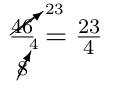# LaTeX fractions

StartLaTeX Topics

## LaTeX fractions

Fractions belong to the standard applications within mathematics, but they are also occasionally used within normal text.

## 1.1 LaTeX fraction in math

Fractions are made with the command \frac{numerator}{denominator}. Example for fractions:
 Input Output $\frac{1}{a}$ $\frac{1}{a}$ $\frac{1}{\frac{a}{b}}$ $\frac{1}{\frac{a}{b}}$ $\frac{\frac{a}{b+c}+d}{a +\frac{b}{c+d}}$ $\frac{\frac{a}{b+c}+d}{a +\frac{b}{c+d}}$ $\frac{1}{a}$ $\frac{1}{a}$ $\frac{1}{\frac{a}{b}}$ $\frac{1}{\frac{a}{b}}$ $\frac{\frac{a}{b+c}+d}{a +\frac{b}{c+d}}$ $\frac{\frac{a}{b+c}+d}{a +\frac{b}{c+d}}$

### 1.1.1 change size of fractions

To display a fraction larger or smaller, the following commands are available from the package amsmath.sty.
 name/command input output displaystyle $\dfrac{1}{\dfrac{a}{b}}$ $\dfrac{1}{\dfrac{a}{b}}$ textstyle $\tfrac{1}{\tfrac{a}{b}}$ $\tfrac{1}{\tfrac{a}{b}}$ scriptstyle ${\frac{1}{\frac{a}{b}} }$ $\frac{1}{\frac{a}{b}}$ scriptscriptstyle ${\scriptscriptstyle \frac{1}{\frac{a}{b}} }$ $\frac{1}{\frac{a}{b}}$

For the latter two sizes scriptstyle and scriptscriptstyle amsmath.sty provides no shortcut commands for these. However, these commands are quite easy to create.

\newcommand{\sfrac}{\genfrac{}{}{}2}
\newcommand{\ssfrac}{\genfrac{}{}{}3}
 $\sfrac{1}{\sfrac{a}{b}}$ $\frac{1}{\frac{a}{b}}$ $\ssfrac{1}{\ssfrac{a}{b}}$ $\scriptscriptstyle \frac{1}{\frac{a}{b}}$

### 1.1.2 LaTeX continued fraction

Normal continued fraction within $environment:$\frac{1}{1+\frac{1}{1+\frac{1}{1+\frac{1}{1 + \frac{1}{1 + x}}}}}$Output:  $\frac{1}{1+\frac{1}{1+\frac{1}{1+\frac{1}{1 + \frac{1}{1 + x}}}}}$ Normal continued fraction within displaymath environment: $\frac{1}{1+\frac{1}{1+\frac{1}{1+\frac{1}{1 + \frac{1}{1 + x}}}}}$ Output: $\frac{1}{1+\frac{1}{1+\frac{1}{1+\frac{1}{1 + \frac{1}{1 + x}}}}}$ Normal continued fraction by using the \cfrac from amsmath.sty within$ environment:
$\cfrac{1}{1+\cfrac{1}{1+\cfrac{1}{1+\cfrac{1}{1 + \cfrac{1}{1 + x}}}}}$
Output:
 $\cfrac{1}{1+\cfrac{1}{1+\cfrac{1}{1+\cfrac{1}{1 + \cfrac{1}{1 + x}}}}}$

Normal continued fraction by using the \cfrac from amsmath.sty within displaymath environment:
$\cfrac{1}{1+\cfrac{1}{1+\cfrac{1}{1+\cfrac{1}{1 + \cfrac{1}{1 + x}}}}}$

Output:
$\cfrac{1}{1+\cfrac{1}{1+\cfrac{1}{1+\cfrac{1}{1 + \cfrac{1}{1 + x}}}}}$

### 1.1.3 LaTeX fractions without line or slash

With the command \substack{numerator \\ denominator} you will be able to set a fraction without slash.
$\substack{a\\b}$
 $\substack{a\\b}$

It will also work within displaymath environment:
$\substack{a\\b}$
$\substack{a\\b}$

### 1.1.4 Parenthesis around fraction

If you want to clamp fractions, you should use the \left and rightvariants.
Without left and right:
$( \frac{a^{2}}{2} )$
Output:
 $( \frac{a^{2}}{2} )$

With left and right:
$\left( \frac{a^{2}}{2} \right)$

Output:
 $\left( \frac{a^{2}}{2} \right)$

## LaTeX fractions within text

In principle, a fraction, such as 12 can also be written in normal text, for example with $\tfrac{1}{2}$. Alternatively, there are separate commands for selected fractions, such as for a quarter:\textonequarter{} ¼ and a half: \textonehalf{} ½.

## usepackage for fractions

The first package nicefrac.sty serves the better representation in the text. The second package cancel.sty enables the simplifying (reducing) of fractions.

### 1.3.1 nicefrac usepackage

After you include the package nicefrac the command \nicefrac{numerator}{denominator} is available. And the example sentence looks much nicer, because the fraction is written in the slanting way known for text. In principle, a fraction, such as ½ can also be written in normal text, for example with $\nicefrac{1}{2}$.

### 1.3.2 cancel usepackage

The cancel.sty usepackage contains the following four commands for simplifying (reducing) of fractions.
 command example description $\cancel{24}$ $\cancel{24}$ Stroke / Line from bottom left to top right $\bcancel{24}$ $\bcancel{24}$ Stroke / Line from top left to bottom right $\xcancel{24}$ $\xcancel{24}$ Two crossing strokes (combination of the first two commands) $\cancelto{23}{46}$ $\cancelto{23}{46}$ Reducing to ...
Input:
\frac{\cancel{24}}{\cancel{8}} = 3$\\ \frac{\cancel{24}}{\bcancel{8}} = 3$\\
\frac{\xcancel{24}}{\bcancel{8}} = 3$\\ \frac{\cancelto{23}{46}}{\cancelto{4}{8}} = \frac{23}{4}$\\
Output:
$\frac{\cancel{24}}{\cancel{8}} = 3$ $\frac{\cancel{24}}{\bcancel{8}} = 3$ $\frac{\xcancel{24}}{\bcancel{8}} = 3$ $\frac{\cancelto{23}{46}}{\cancelto{4}{8}} = \frac{23}{4}$ The first three commands also work with text:
 Input Output \cancel{Text} $\cancel{\text{Text}}$ \bcancel{Text} $\bcancel{\text{Text}}$ \xcancel{Text} $\xcancel{\text{Text}}$
The representation of the cancelto command can be improved a little bit by hand:instead ofTherefore the command cancelto would be changed to:
\newcommand{\bcancelto}{\rotatebox[origin=c]{180}{$\cancelto{\rotatebox[origin=c]{180}{\scriptsize #1}}{#2}$}}

Hint: The command rotatebox is part of the graphicx.sty usepackage, therefore you have to include this usepackage too.

Input:
$\frac{\cancelto{23}{46}}{\bcancelto{4}{8}} = \frac{23}{4} \text{ instead of } \frac{\cancelto{23}{46}}{\cancelto{4}{8}} = \frac{23}{4}$

Output:instead of